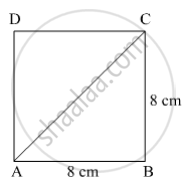SSC (English Medium) Class 8Maharashtra State Board
Share

# Find the Length of Diagonal of a Square with Side 8 Cm. - SSC (English Medium) Class 8 - Mathematics

ConceptSome Special Parallelograms Concept of a Square

#### Question

Find the length of diagonal of a square with side 8 cm .

#### Solution

Suppose ABCD is a square of side 8 cm.Here, segment AC is a diagonal of square ABCD.

In ∆ABC,

l(AC)2 = l(AB)+ l(BC)2               (Pythagoras theorem)

⇒ l(AC)= (8)2 + (8)2

⇒ l(AC)= 64 + 64 = 128

⇒ l(AC) = $\sqrt{128}$ cm

Thus, the length of diagonal of square is $\sqrt{128}$ cm .

Is there an error in this question or solution?

#### APPEARS IN

Balbharati Solution for Balbharati Class 8 Mathematics (2019 to Current)
Chapter 8: Quadrilateral : Constructions and Types
Practice Set 8.2 | Q: 6 | Page no. 47
Solution Find the Length of Diagonal of a Square with Side 8 Cm. Concept: Some Special Parallelograms - Concept of a Square.
S